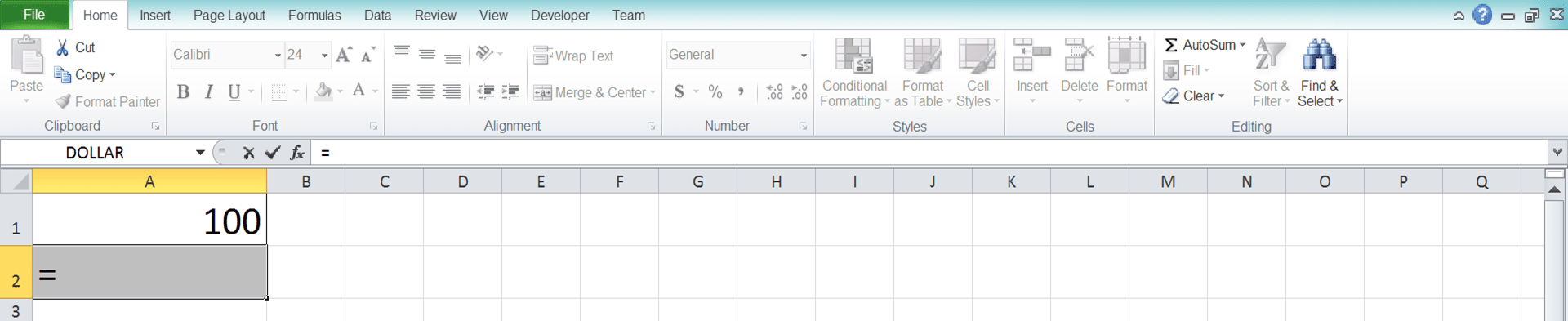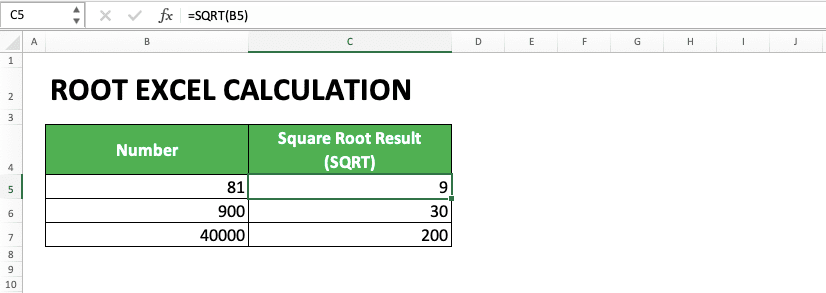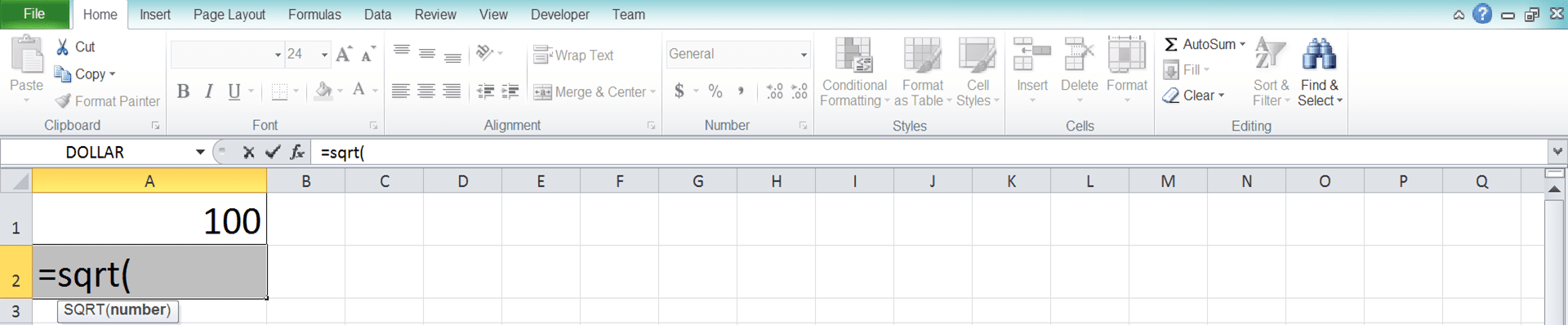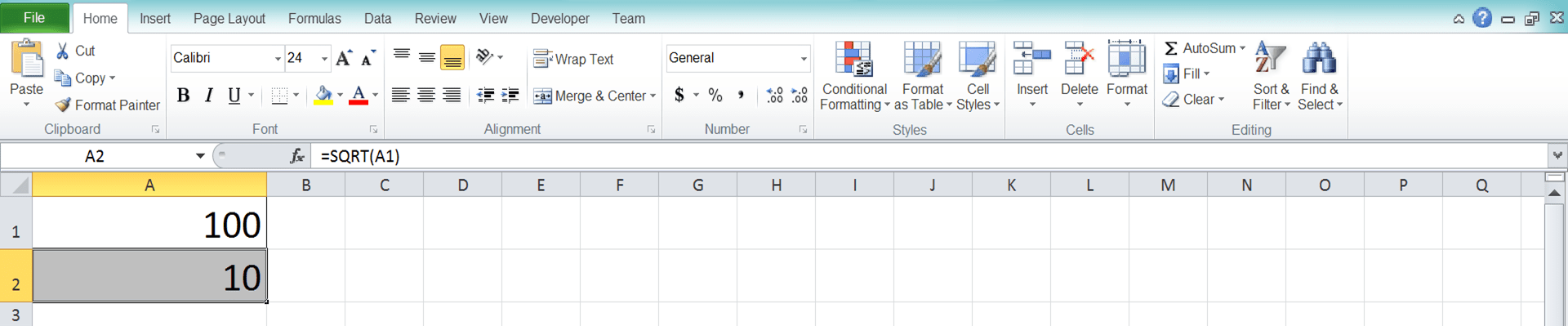Root Excel Calculation and All Its Formulas & Functions - Compute Expert

# Root Excel Calculation and All Its Formulas & Functions

Home >> Excel Tutorials from Compute Expert >> Excel Calculations >> Root Excel Calculation and All Its Formulas & Functions

In this tutorial, you will learn completely how to write a root formula and calculate root in excel. The excel root calculation is one of the important things to learn if we want to process our numeric data optimally.

This tutorial will begin with the introduction of three methods you can use to calculate root in excel (manually and using built-in formulas). After that, we will discuss how to calculate square, cubic, and n-th root in excel using those three methods. Then, we will give an exercise you can do to sharpen your understanding and also an additional note about this calculation.

If you just want to learn a part of the tutorial, you can do that too. Just jump to that part directly by clicking it from the table of contents below.

Disclaimer: This post may contain affiliate links from which we earn commission from qualifying purchases/actions at no additional cost for you. Learn more

## Method 1: Write a Root Formula in Excel Manually (With the Help of the ^ Symbol)

To calculate root in excel, one method you can use is by utilizing the caret symbol (^) in your keyboard. This symbol actually represents the power calculation in excel. However, because the root is the opposite of power, you can use it for root calculation too. This is done by adding “1/“ in the power level input.

To make the explanation clearer, here is a general illustration of the manual root calculation writing in excel:

=number^(1/level)

The inputs you need to give for the writing can be explained briefly as follows:
• number = the number you want to calculate the root of
• level = the level of your root calculation

Thus, for example, you want to calculate the fifth root of 32, then input in your cell: =32^(1/5). If you want to calculate the third root of 125, then the writing is = 125^(1/3). You just need to follow that pattern so you can write your root formula in excel manually!

The example of manual writing and its result in excel can be seen below.In the example’s formula bar, we can see how the writing of the root formula is done with the ^ symbol. You just need to input your number, ^ symbol, “1/“, and your root calculation level. Press enter and the root calculation result you want will be there!

To make it clearer about how to use this method, the following will give detailed step-by-step writing. Each step will be explained with a screenshot to help you understand it easier.

1. Type an equal sign ( = ) in the cell where you want to put the root calculation result2. Input the number or cell coordinate where the number that you want to calculate the root is. Then, type a caret sign ( ^ ) and an open bracket sign3. Type 1 and a slash sign ( / ) as the opposite symbol of the power calculation4. Input the root level or the cell coordinate where the number you want to make as the root level is5. Type a close bracket sign6. Press Enter
7. The process is done!## Method 2: Use the POWER Formula

Besides the manual writing method using the ^ symbol, you can also use the POWER formula for your root calculation. As with the ^ symbol, the POWER original function is to do the power calculation in excel. But because the root is the opposite of power, then this formula can also be used for the root calculation.

Generally, the POWER formula writing in excel to do the root calculation can be illustrated as follows.

=POWER(number, 1/level)

The explanation about the inputs used in the writing above is:
• number = the number you want to calculate the root of
• level = the level of your root calculation

The “1/“ writing there, just as in the manual writing method, is to change the power level into a root level. Therefore, don’t forget to input your root level after typing the “1/“ first.

The example of POWER writing and its root calculation result in excel can be seen in the screenshot below.As you can see in the screenshot, POWER is used to calculate the root of a number accurately. Make sure you input the number you want to calculate, “1/“, and the root level correctly. You will get your root calculation result in no time!

To make it clearer for your understanding of the POWER use, you can see the step-by-step writing below.

1. Type an equal sign ( = ) in the cell where you want to put the root calculation result2. Type POWER (can be with large and small letters) and an open bracket sign after =3. Input the number or the cell coordinate where the number that you want to calculate the root is. Then, type a comma sign ( , )4. Type 1 and a slash sign ( / ) as the reversal symbol of the power process in POWER into a root process5. Input the root level or the cell coordinate where the number you want to make as the root level is6. Type a close bracket sign7. Press Enter
8. The process is done!## Method 3: Use the SQRT Formula (Just for the Square Root Calculation)

If what you need is a square root result, then there is a formula to get that fast. That formula is the SQRT formula.

Generally, SQRT writing can be illustrated as follows:

=SQRT(number)

As probably felt when looking at its above writing, SQRT is pretty easy to use because it only needs one input. That input is the number you want to square root.

To illustrate how easy to use SQRT is, see its usage example’s screenshot below.As you can see in the example’s formula bar, you just need to input your number in the SQRT formula. Press enter and the square root of your number will be there in your cell!

To make the SQRT writing method clearer, here will be given the steps to write the formula in excel.

1. Type an equal sign ( = ) in the cell where you want to put your square root result2. Type SQRT (can be with large and small letters) and an open bracket sign after =3. Input the number or the cell coordinate where the number you want to square root is, after the open bracket sign4. Type a close bracket sign5. Press Enter
6. The process is done!## Square Root Calculation in Excel

The easiest way to do a square root calculation in excel is, as mentioned earlier, by using the SQRT formula. You just need to input your number in the formula and you will get your result immediately.

The SQRT formula writing for the square root calculation in excel is as follows:

=SQRT(number_to_square_root)

You can also use POWER or the manual writing method if you want. The writing of those two methods to calculate the square root in excel is as follows:

Manual (with the ^ symbol):

=number_to_square_root^(1/2)

POWER:

=POWER(number_to_square_root,1/2)

Because the square root uses 2 as its root level, we need to input 2 in our root level input part.

Here is the usage and result example of SQRT, manual writing, and POWER when they do square root calculations in excel.As you can see, SQRT, manual writing, and POWER can be used to square root and they give the same results. As long as their inputs are given correctly, they can square root your number depending on your data processing needs.

## Cube Root Calculation in Excel

How about the cube root calculation using excel? There are two ways to do it which is manual with the ^ symbol and by using the POWER formula.

Both writing to calculate the cube root of a number can be illustrated as follow:

Manual (with the ^ symbol):

=number_to_cube_root^(1/3)

POWER:

=POWER(number_to_cube_root,1/3)

Because the cube root level is 3, we input 3 in the appropriate input part of the two methods. Don’t forget to input “1/“ in front of the 3 so the root calculation can be done correctly.

The usage and result example of the two cube root calculation methods is as follows.As the example shows, the two cube root calculation methods give the same results as long as they are written correctly.

## Nth Root Calculation in Excel

The nth root calculation in excel can be done by using the manual writing method and the POWER formula.

Both writings for the calculation can be illustrated as follow:

Manual (with the ^ symbol):

=number_to_root^(1/n)

POWER:

=POWER(number_to_root,1/n)

n in both writing illustrations is the root level you want to use in your calculation process.

The usage and result example of both methods is as follows.As you can see, the writing format of both methods is the same as described earlier. With the writing, each of them will give the root calculation result you need from a number.

## Exercise

After you have learned how to calculate root in excel and its formulas, now let’s do an exercise. This exercise is done so you can practice your understanding so you can be more adept at doing this root calculation!

### Questions

1. What is the SQRT result for the number?
2. What is the result if the level is 5? Use the manual or POWER formula method!

Usually, the root calculation result of your number is decimal and you most probably need to round it. If you need to do this, learn this tutorial if you haven’t understood how.

Related tutorials you should learn:

Get updated excel info from Compute Expert by registering your email. It's free!